17.3 连续变量的无向图模型¶

weiya 注：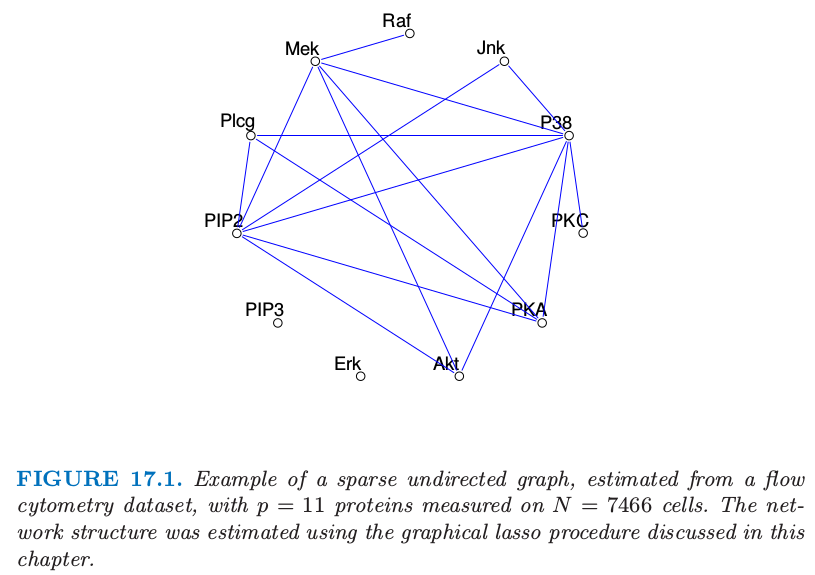weiya 注：Ex. 17.3

• \eqref{17.6} 中的 $Y$ 对 $Z$ 的依懒性只与均值项有关．显然，$\beta$ 中的 $0$ 元素，也是 $\theta_{ZY}$ 中的 $0$ 元素，意味着 $Z$ 中的对应元素与 $Y$ 在给定其余变量的条件下是独立的．
• 我们可以通过多重线性回归学习这个依赖性结构．

图结构已知时参数的估计¶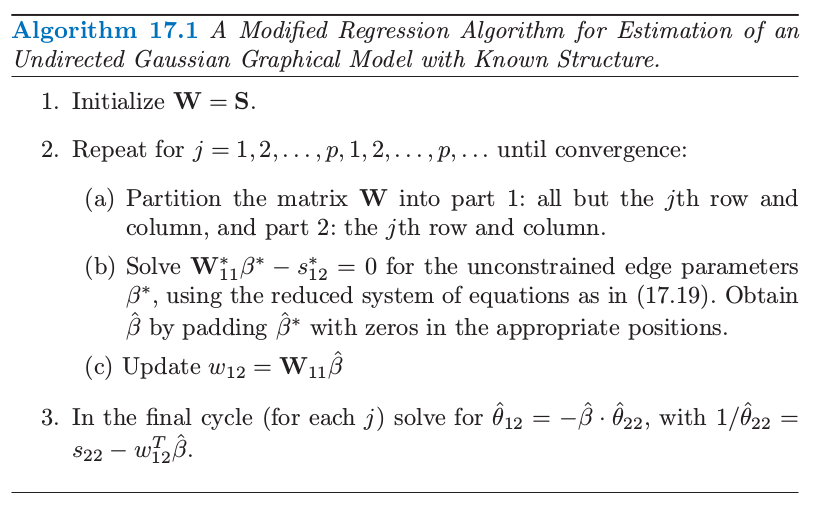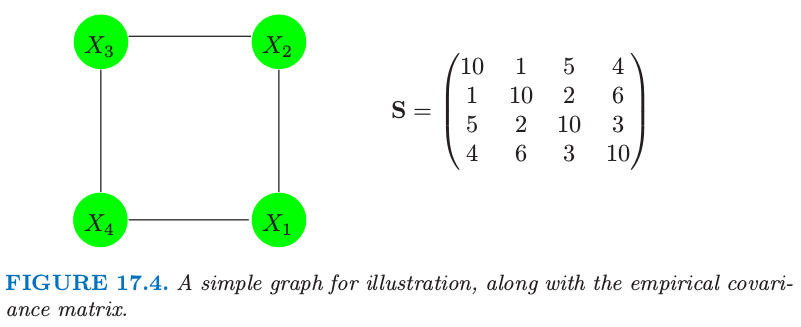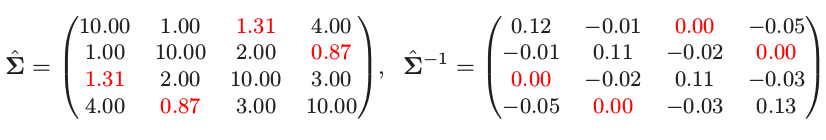weiya 注：

图结构的估计¶

weiya 注：

Meinshausen and Bühlmann (2006)9 对这个问题采取简单的方式：不是试图完全估计 $\mathbf \Sigma$ 或者 $\mathbf \Theta=\mathbf \Sigma^{-1}$，他们仅仅估计非零的组分 $\theta_{ij}$．为了实现这点，它们将每个变量看成响应变量而其它的变量作为预测变量进行拟合 lasso 回归．如果变量 $i$ 在变量 $j$ 上的估计系数为非零，或者（并且）$j$ 变量在 $i$ 上的估计系数为非零，则组分 $\theta_{ij}$ 估计为非零．它们证明这个过程渐近地一致估计了 $\mathbf\Theta$ 的非零元的集合．

weiya 注：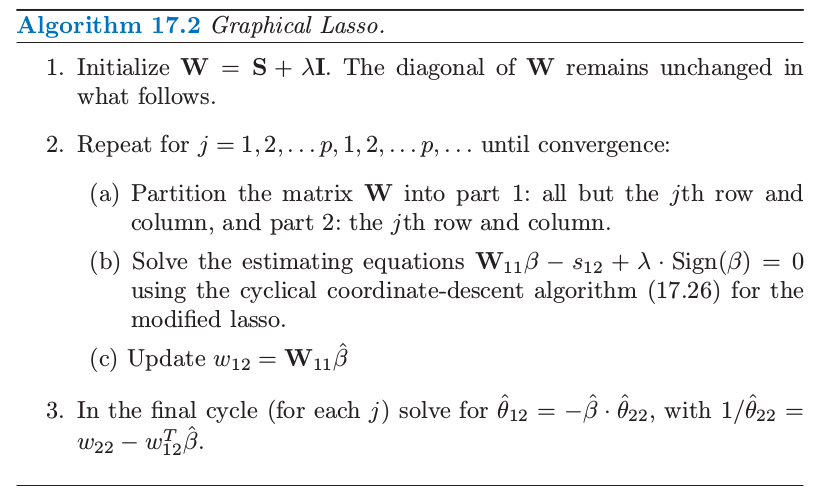Friedman et al. (2008b)9 采用成对坐标下降方法（3.8.6 节）来在一步求解修改的 lasso 问题．下面是图 lasso 算法的成对坐标下降细节．令 $\mathbf {V=W_{11}}$，更新式有如下形式 $j=1,2,\ldots,p-1,1,2,\ldots,\ldots,p-1,\ldots$，其中 $S$ 为软阈限算子 这个过程对预测变量循环直到收敛．

Graphical lasso 算法非常快，可以在一分钟之内求解含 1000 个结点的中等稀疏的问题．可以很简单地修改算法得到特定边的惩罚参数 $\lambda_{jk}$；因为 $\lambda_{jk}=\infty$ 会强制使 $\hat\theta_{jk}$ 为 0，这个算法归入到算法 17.1 中．通过将稀疏逆协方差矩阵问题作为一系列回归，可以快速地计算并且验证解的路径作为惩罚参数$\lambda$ 的函数．更多的细节可以在 Friedman et al. (2008b)9 中找到．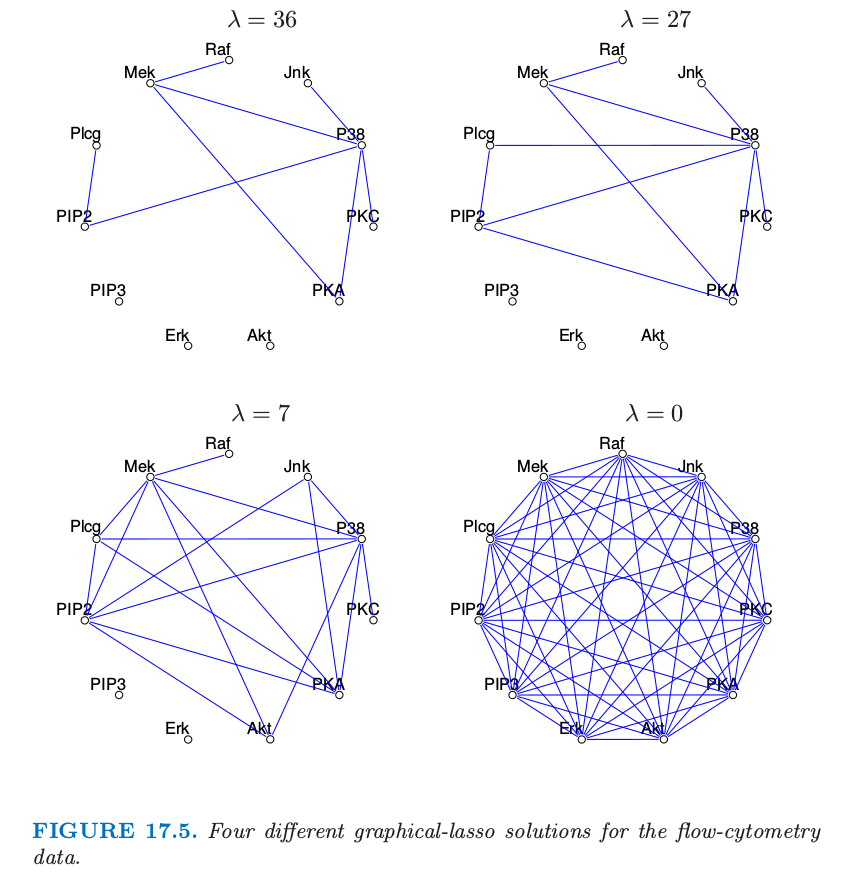weiya 注: Ex. 17.9

1. Mardia, K., Kent, J. and Bibby, J. (1979). Multivariate Analysis, Academic Press.

2. Butte, A., Tamayo, P., Slonim, D., Golub, T. and Kohane, I. (2000). Discovering functional relationships between RNA expression and chemotherapeutic susceptibility using relevance networks, Proceedings of the National Academy of Sciences pp. 12182–12186.

3. Chaudhuri, S., Drton, M. and Richardson, T. S. (2007). Estimation of a covariance matrix with zeros, Biometrika 94(1): 1–18.

4. Speed, T. and Kiiveri, H. T. (1986). Gaussian Markov distributions over finite graphs, Annals of Statistics 14: 138–150.

5. Whittaker, J. (1990). Graphical Models in Applied Multivariate Statistics, Wiley, Chichester.

6. Lauritzen, S. and Spiegelhalter, D. (1988). Local computations with probabilities on graphical structures and their application to expert systems, J. Royal Statistical Society B. 50: 157–224.

7. Boyd, S. and Vandenberghe, L. (2004). Convex Optimization, Cambridge University Press. 下载

8. Dempster, A. (1972). Covariance selection, Biometrics 28: 157–175.

9. Friedman, J., Hastie, T. and Tibshirani, R. (2008b). Sparse inverse covariance estimation with the graphical lasso, Biostatistics 9: 432–441.

10. Banerjee, O., Ghaoui, L. E. and d’Aspremont, A. (2008). Model selection through sparse maximum likelihood estimation for multivariate gaussian or binary data, Journal of Machine Learning Research 9: 485–516.